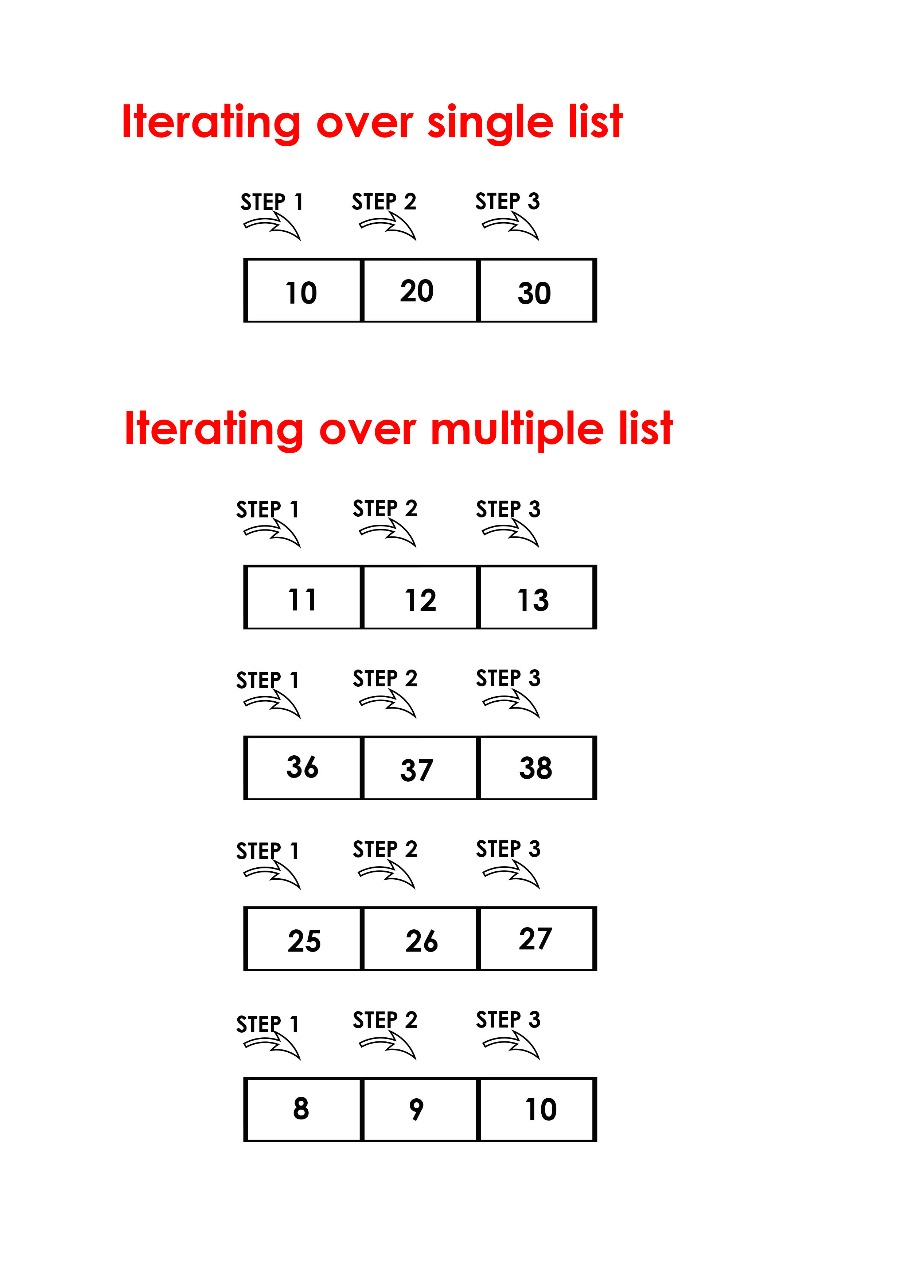# Python | Iterate over multiple lists simultaneously

• Difficulty Level : Easy
• Last Updated : 18 Aug, 2021

Iterating over single lists, refers to using for loops for iteration over a single element of a single list at a particular step whereas in iterating over multiple lists simultaneously, we refer using for loops for iteration over a single element of multiple lists at a particular step.Iterate over multiple lists at a time

For better understanding of iteration of multiple lists, we are iterating over 3 lists at a time.

We can iterate over lists simultaneously in ways:

1. zip() : In Python 3, zip returns an iterator. zip() function stops when anyone of the list of all the lists gets exhausted. In simple words, it runs till the smallest of all the lists.

Below is an implementation of the zip function and itertools.izip which iterates over 3 lists:

## Python3

 `# Python program to iterate``# over 3 lists using zip function`` ` `import` `itertools `` ` `num ``=` `[``1``, ``2``, ``3``]``color ``=` `[``'red'``, ``'while'``, ``'black'``]``value ``=` `[``255``, ``256``]`` ` `# iterates over 3 lists and executes ``# 2 times as len(value)= 2 which is the``# minimum among all the three ``for` `(a, b, c) ``in` `zip``(num, color, value):``     ``print` `(a, b, c)`

Output:

```1 red 255
2 while 256
```
2. itertools.zip_longest() : zip_longest stops when all lists are exhausted. When the shorter iterator(s) are exhausted, zip_longest yields a tuple with None value.

Below is an implementation of the itertools.zip_longest which iterates over 3 lists:

## Python3

 `# Python program to iterate``# over 3 lists using itertools.zip_longest`` ` `import` `itertools `` ` `num ``=` `[``1``, ``2``, ``3``]``color ``=` `[``'red'``, ``'while'``, ``'black'``]``value ``=` `[``255``, ``256``]`` ` `# iterates over 3 lists and till all are exhausted``for` `(a, b, c) ``in` `itertools.zip_longest(num, color, value):``    ``print` `(a, b, c)`

Output:

```1 red 255
2 while 256
3 black None
```

Output:

```1 red 255
2 while 256
3 black None
```

We can also specify a default value instead of None in zip_longest()

## Python3

 `# Python program to iterate``# over 3 lists using itertools.zip_longest`` ` `import` `itertools `` ` `num ``=` `[``1``, ``2``, ``3``]``color ``=` `[``'red'``, ``'while'``, ``'black'``]``value ``=` `[``255``, ``256``]`` ` `# Specifying default value as -1``for` `(a, b, c) ``in` `itertools.zip_longest(num, color, value, fillvalue``=``-``1``):``    ``print` `(a, b, c)`

Output:

```1 red 255
2 while 256
3 black -1
```

Note :
Python 2.x had two extra functions izip() and izip_longest(). In Python 2.x, zip() and zip_longest() used to return list, and izip() and izip_longest() used to return iterator. In Python 3.x, there izip() and izip_longest() are not there as zip() and zip_longest() return iterator.

My Personal Notes arrow_drop_up